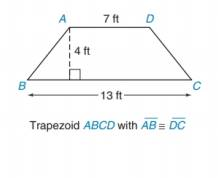Chapter 8.2, Problem 13E### Elementary Geometry for College St...

6th Edition
Daniel C. Alexander + 1 other
ISBN: 9781285195698

#### Solutions

Chapter
Section### Elementary Geometry for College St...

6th Edition
Daniel C. Alexander + 1 other
ISBN: 9781285195698
Textbook Problem
1 views

# In Exercises 13 to 18, find the area of the given polygon.To determine

To Find:

The area of the trapezium.

Explanation

Formula Used:

The area A of a trapezoid whose bases have lengths b1 and b2 and whose altitude has length h is given by A=12h(b1+b2)

Calculation:

It is given that the trapezium ABCD with AB¯DC¯. Therefore the given trapezium is an isosceles trapezium.

The two parallel bases of the trapezoids are AD=7 ft and BC=13 ft with the altitude AE and DF as 4 ft.

### Still sussing out bartleby?

Check out a sample textbook solution.

See a sample solution

#### The Solution to Your Study Problems

Bartleby provides explanations to thousands of textbook problems written by our experts, many with advanced degrees!

Get Started